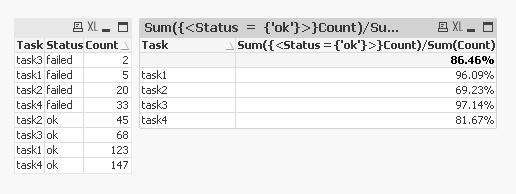# QlikView Creating Analytics

Discussion Board for collaboration related to Creating Analytics for QlikView.

HighlightedNot applicable

## Calculate ratio with measurements on multiple rows

Hi everyone,

I'm very new on QlikSense but already loving it !

I think I have missed something in the way of building expressions, or maybe what I want to do is not possible, but I can't find a way to do calculate my percentage. Here is an example :

As you can see, each task can have 2 statuses which can be "failed" or "ok".

I want to get the success rate of each task by calculating the ratio of successful vs failed tasks.

Thanks in advance for any help !

Tags (3)
1 Solution

Accepted SolutionsMVP

## Re: Calculate ratio with measurements on multiple rows

Is this what you want?10 RepliesMVP

## Re: Calculate ratio with measurements on multiple rows

successful vs failed or successful vs overall?

Successful vs failed:

Sum({<Status = {'ok'}>}Count)/Sum({<Status = {'failed'}>}Count)

Successful vs overall:

Sum({<Status = {'ok'}>}Count)/Sum(Count)

Valued Contributor III

## Re: Calculate ratio with measurements on multiple rowsNot applicable

## Re: Calculate ratio with measurements on multiple rows

Hi,

I tryed your expression, adding a measure to my simple table, but only the total ration is calculated. All the other rows have 0 value, and I need this ratio for each task.MVP

## Re: Calculate ratio with measurements on multiple rows

Is this what you want?Not applicable

## Re: Calculate ratio with measurements on multiple rows

Yes perfect ! Sorry I did not understand that I needed to make an other table.

Now I understand far better how it works !

Thank you for your amazing help !MVP

## Re: Calculate ratio with measurements on multiple rows

No problem at allNot applicable

## Re: Calculate ratio with measurements on multiple rows

is there a way to get ratio as non %?MVP

## Re: Calculate ratio with measurements on multiple rows

Not sure if I understand your question?Not applicable

## Re: Calculate ratio with measurements on multiple rows

for ex:

if i want the ratio from start to finish .

start:     finish

3          6

5          10

20          100

i know how to use expression to get % but i want to show as 3:6 which simplifies to 1:3,  and 5:10 will be 1:5 and 20:100 will be 5:25

thanks